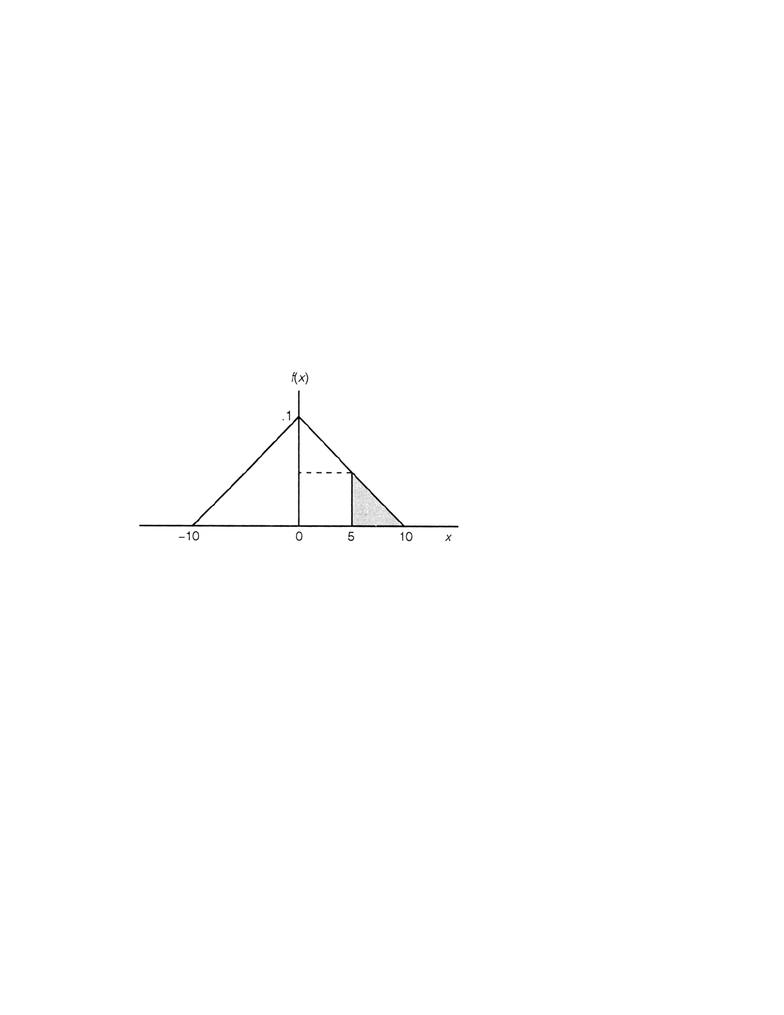# OMIS 2010 Chapter Notes - Chapter 08: Standard Normal Deviate, Random Variable, Exponential Distribution

21 views13 pagesChapter 8: Continuous Probability Distributions
8.1 Introduction
This chapter continued our discussion of probability distributions. It began by describing continuous
probability distributions in general, and then took a detailed look at the normal distribution, which is the
most important specific continuous distribution. At the completion of this chapter, you are expected to
understand the following:
1. The basic differences between discrete and continuous random variables.
2. How to convert a normal random variable into the standard normal random variable, and how to
use the table of standard normal probabilities.
3. How to recognize when it is appropriate to use an exponential distribution, and how to compute
exponential probabilities.
8.2 Continuous Probability Distributions
This section introduced the notion of a continuous random variable, which differs from a discrete
random variable both in the type of numerical events of interest and the type of function used to find
probabilities. A continuous random variable X can assume any value in some interval, such as 5 < x <
20. Since a continuous random variable can assume an uncountably infinite number of values, the
probability that any particular value will be assumed is zero. Hence, for a continuous random variable X,
it is only meaningful to talk about the probability that X will assume a value within a particular interval.
Such a probability is found using the probability density function, f(x), associated with X. The
probability that X will take a value in the interval a < x < b is given by the area under the graph of f(x)
between the values a and b. For most of the specific continuous distributions that you will encounter
(such as the normal distribution), you can easily compute probabilities such as this by using probability
tables appearing in the appendices. Notice that because
P(X = a) = P(X = b) = 0
the following equality holds for any continuous random variable X:
P(a < X < b) = P(a X b)
A specific continuous distribution that is especially simple to work with is the uniform
distribution. A random variable X defined over an interval a x b is uniformly distributed if its
probability density function is given by
f(x) = 1
ba, a x b
93
Unlock document

This preview shows pages 1-3 of the document.
Unlock all 13 pages and 3 million more documents.Because the graph of the density function is a horizontal line, the area under the graph is a rectangle,
giving rise to an alternative name for the uniform distribution—the rectangular distribution. Notice that
the values of a uniform random variable X are distributed evenly, or uniformly, across the domain of X.
Example 8.1
A continuous random variable X has the following probability density function:
f(x)=.01
x
+.1 for
10
x
0
.01x+.1 for 0 x10
a) Graph the density function f(x).
b) Verify that f(x) satisfies the requirements of a probability density function.
c) Find P(X 5).
Solution
a)
b) To verify that f(x) is indeed a probability density function, we observe that:
1. f(x) 0 for all values of x.
2. The total area under the graph of f(x) is 1. To see this, recall that the area of a triangle is
bh/2, where b is the base and h is the height of the triangle. Therefore, the area under f(x) to
the right of x = 0 is (10)(.1)/2 = .5. Due to symmetry, the area under f(x) to the left of x = 0
is also .5, so the total area under f(x) is .5 + .5 = 1.
c) To find P(X 5), we must find the area under the graph of f(x) to the right of x = 5. This area is
the shaded triangle shown on the graph in part a). The base of this triangle is (10 – 5) = 5, and
the height is f(5) = –.01(5) + .1 = .05. Hence,
P(X 5) = (5)(.05)/2 = .125
94
Unlock document

This preview shows pages 1-3 of the document.
Unlock all 13 pages and 3 million more documents.EXERCISES
8.1 Consider a uniform random variable X with the following probability density function:
f(x) = 1
50, 20 x 70
a) Graph the density function f(x).
b) Verify that f(x) satisfies the requirements of a probability density function.
c) Find P(X 40).
d) Find P(X 39).
8.2 A continuous random variable X has the following probability density function:
f(x) = –.08x + .4, 0 x 5
a) Graph the density function f(x).
95
Unlock document

This preview shows pages 1-3 of the document.
Unlock all 13 pages and 3 million more documents.

## Document Summary

This chapter continued our discussion of probability distributions. It began by describing continuous probability distributions in general, and then took a detailed look at the normal distribution, which is the most important specific continuous distribution. This section introduced the notion of a continuous random variable, which differs from a discrete random variable both in the type of numerical events of interest and the type of function used to find probabilities. Hence, for a continuous random variable x, it is only meaningful to talk about the probability that x will assume a value within a particular interval. Such a probability is found using the probability density function, f(x), associated with x. The probability that x will take a value in the interval a < x < b is given by the area under the graph of f(x) between the values a and b.